# Syllogisms Solutions

The answers to the Syllogism Questions are given along with their respective Venn diagrams. The solutions are easy to understand and are sure to effectuate the CAT preparation.

1. (B). Only (B) conclusion follows

Solution: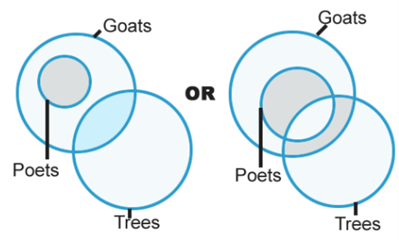2. (D). Neither (A) nor (B) follows

Solution: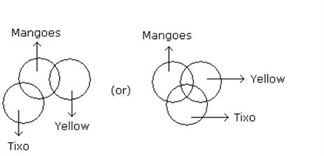3. (B). Only (B) conclusion follows

Solution: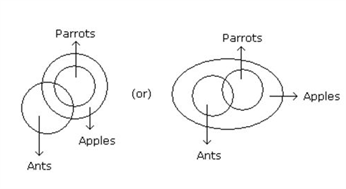4. (E). Both (A) and (B) follow.

Solution: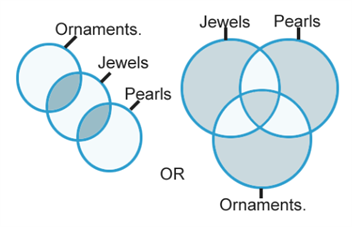5. (E). Both (A) and (B) follow.

Solution: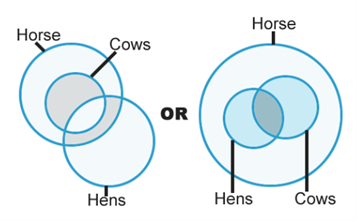6. (B). Only (B) conclusion follows

Solution: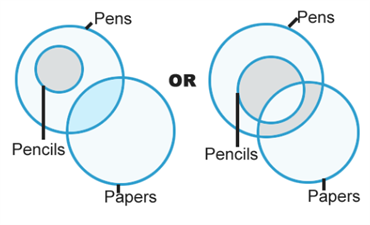7. (E). Both (A) and (B) follow.

Solution: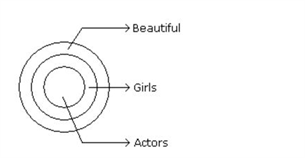8. (D). Neither (A) nor (B) follows

9. (B). Only (B) conclusion follows

Solution: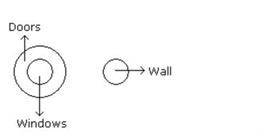10. (B). Only (B) conclusion follows

Solution: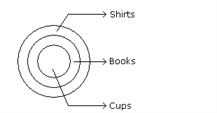11. (D). Neither (A) nor (B) follows.

12. (E). Both (A) and (B) follow.

Solution:13. (D). Neither (A) nor (B) follows

Solution: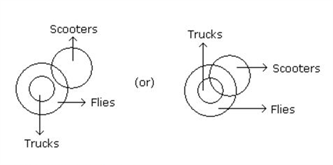14. (A). Only (A) conclusion follows

Solution: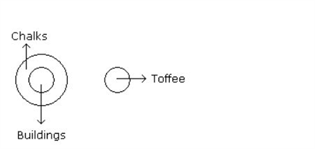15. (D). Neither (A) nor (B) follows.

16. (D). Only (C)

Solution: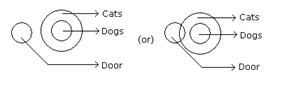17. (A). Only (A) and (B)

Solution: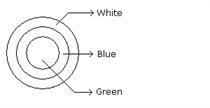18. (C). Only (C)

Solution: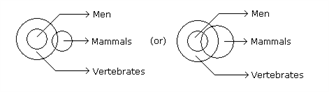19. (C). Only (B) and (D)

Solution: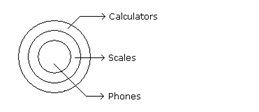20. (D). Only (D).

21. (E). None of the four.

Solution: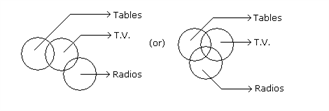22. (D). None of the four.

Solution: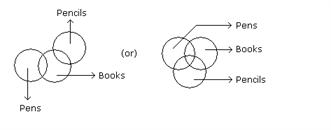23. (C). Only (A), (C) and (D)

Solution: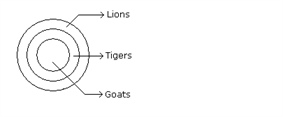24. (A). Only (C).

Solution: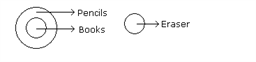25. (A). Only (C) and (D).

Solution: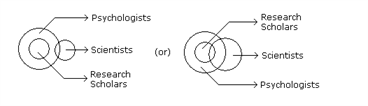26. (B). Only (A).

Solution: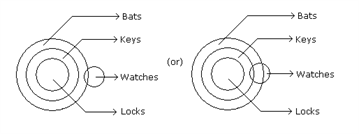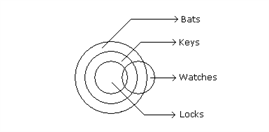27. (D). Only (A) and (D).

Solution: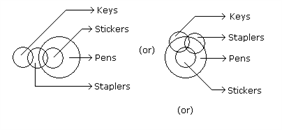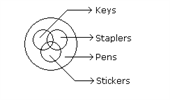28. (B). Only (A) and (D).

Solution: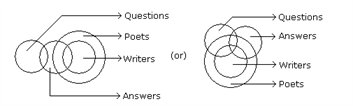29. (B). Only (C).

Solution: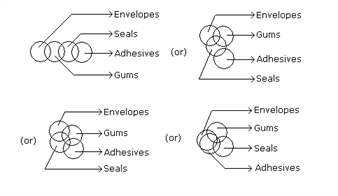30. (A). Only (A).

Solution: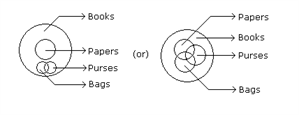31. (E). Only (C).

32. (E). None of the four.

Solution: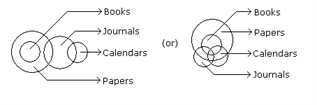33. (A). Only (C) and (D).

Solution: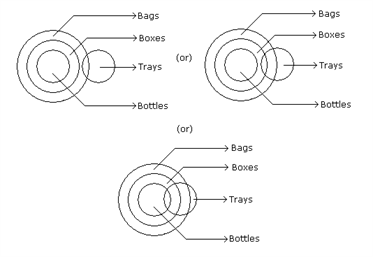34. (A). None of three.

Solution: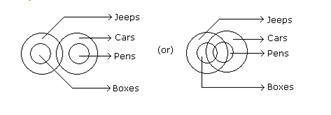Click syllogism to know more about the topic and learn to solve the related questions with ease. To know get more important sample questions for the CAT exam, stay tuned with BYJU’S. Also, get the latest notifications, mock questions, syllabus and pattern along with numerous video lessons to effectuate the learning process.